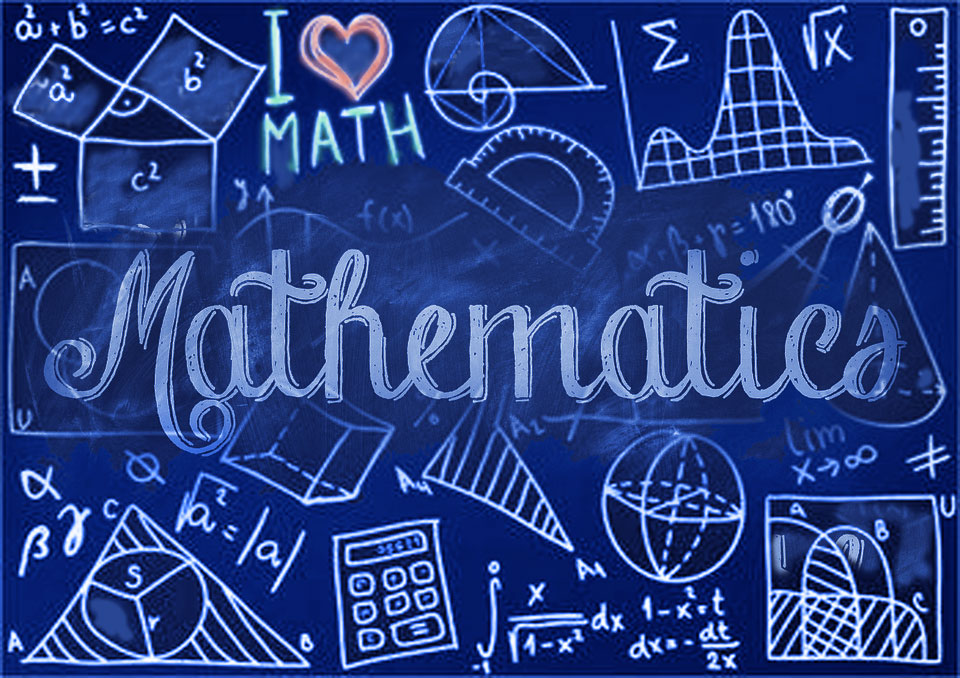Math

Teacher: Kimmara Griffiths

This class serves as a basic introduction to math and the binary operations.  It has been structured to lay a foundation for students to gain “number sense”. Mrs. Griffiths created this class to assist students in mastering basic operations while using several methods to encourage students to have a fluent understanding of math in the “real world”.

ISM Math Level 1 – Arithmetic

Session 1

Approximately 12 Weeks

Whole Numbers

• A history of numbers
• what is zero?
• whole number place value
• Basic computation
• Long Division
• Multi-digit Multiplication Fractions
• The nature of fractions
• Multiplying and dividing fractions
• Mixed numbers/ Improper Fractions

Session 2

Approximately 12 Weeks

Decimal Fractions

• Decimal Place values
• Decimal Word Names
• Operations with decimals
• Converting fractions to decimal
• Rounding review
• Scientific Notation
• Percent
• Using Percent
• Calculating Percent
• Converting to and from percent

Session 3

Approximately 12 Weeks

Measurement/Data

• US/Metric
• Time
• Temperature
• Currency
• Data and Graphing Geometry
• Lines and angles
• Shapes with 2 dimensions in a plane

ISM Math Level 2 – Geometry and Statistics

Session 1

Approximately 12 Weeks

• African influences in Math History
• Multicultural math
• Origin of “zero”
• Operations with integers
• Variables
• Expressions
• Equations
• Inequalities

Focus Activity- Math and the Great Pyramids

Session 2

Approximately 12 Weeks

• Polygons and Patterns
• Angles
• Tessellations
• Islamic Art
• Sequencing and Patterns
• Ethiopian math
• Fibonacci Sequence
• Fractals
• Math as Art

Focus Activity: African Print Fabric

Session 3

Approximately 12 Weeks

• What is percent?
• Calculating Percent
• Data Tables
• Types of Graphs

• Mean
• Median
• Mode
• histograms
• Stem and leaf
• box and whisker plots

Students who successfully complete this course may take a higher-level course.

ISM Math Level 3 – Pre- Algebra

Session 1

Approximately 12 Weeks

Ratios and Proportional Relationship

• Proportional Relationships
• Unit Rate and Constant of Proportionality
• Ratios and Rates Involving Fraction
• Ratios of Scale Drawings

Rational Numbers

• Addition and Subtraction of Integer and Rational Numbers
• Multiplication and Division of Integers and Rational Numbers
• Applying Operations with Rational Numbers to Expressions and Equations
• Math Lab: Unit Pricing

Session 2

Approximately 12 Weeks

Expressions and Equations

• Use Properties of Operations to Generate Equivalent Expressions
• Solve Problems Using Expressions
• Equations, and Inequalities
• Use Equations and Inequalities to Solve Geometry Problems
• Percent and Proportion
• Finding the Whole Percent Problems Including More than One Whole
• Scale Drawing Population, Mixture, and Counting Problems Involving Percent

Session 3

Approximately 12 Weeks

• Geometry
• Unknown Angles
• Triangles
• Slicing Solids
• Area and Surface Area
• Volume Statistics and Probability
• Calculating and Interpreting Probabilities
• Estimating Probabilities
New to homeschooling?